Types of Errors in Hypothesis Testing

Key Terms

o       Type I error

o       Type II error

Objectives

o       Recognize the types of errors that can occur in hypothesis testing

o       Reinforce skills and understanding by way of practice problems

Error and the Significance Level

We accepted the hypothesis that a particular coin was fair because the test statistic for a large data set did not exceed a certain critical value (that is, the number of heads did not vary in a statistically significant sense from the expected value for a fair coin). Nevertheless, we can conceivably imagine a case where it just so happens that a loaded coin "looks" fair in a given set of trials. Although such an occurrence might have a very small probability, it is nonetheless possible. (Alternatively, consider a fair coin: there is a non-zero probability that a series of 1,000 flips comes up all heads! Of course, this is a small probability, but the situation indicates that hypothesis testing does not always produce correct results.)

We can assess the probability of two different types of error for a given significance level. These errors are typically termed Type I and Type II errors. Type I error involves cases where a hypothesis is true, but it is rejected because the test statistic exceeds the critical value for the significance level α. This might be considered a "false negative" result. (The above-mentioned case of a fair coin that so happens by chance to turn up heads an abnormally large number of times, resulting in the rejection of the hypothesis that the coin is fair, is an example of Type I error.) The probability of a Type I error occurring is the same as the significance level--in other words, the probability of a Type I error decreases with decreasing α. Recall that for a random variable (or test statistic) X,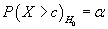Type II error occurs when the null hypothesis is false, but the data does not indicate that it should be rejected. This situation could be considered a "false positive" result. Such a case might involve a loaded coin that happens to have a fair distribution of heads and tails in a certain series of flips. The probability that a Type II error occurs is the same as the probability that the random variable (or test statistic) does not exceed the critical value c if the alternative hypothesis is assumed to be true: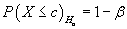The probability β is therefore the probability that a Type II error will not occur.

In light of these errors, we can see that the choice of α (and therefore β) is not purely arbitrary--this choice has a significant effect on whether the probability that the analysis yields a correct result. Although we will not go into further depth on appropriate selection of α (or, perhaps more appropriately, c) such that the probability of errors is minimized, it is important that you remain aware that your choice of α is not without consequence.

Hypothesis Testing Practice Problems

The remainder of this article is devoted to giving you the opportunity to practice your skills on two example problems.

Interested in learning more? Why not take an online Statistics course?

Practice Problem: A type of seed has a germination rate of 95%. For a given packet of 1,000 seeds, 821 of the seeds germinate. Determine if this packet displays a statistically significant deviation from the stated germination rate. Assume that the germination rate for 1,000 seeds follows a normal distribution with a mean of 950 and a standard deviation of 48.

Solution: Let's apply our hypothesis testing procedure. First, we want to formulate our hypotheses:

H0: The germination rate is 95%.

Ha: The germination rate is less than 95%.

Let's use a significance level of α = 0.01. We can define our test statistic X as follows.

X = The number of seeds out of 1,000 that germinate

We are told that the random variable X follows a normal distribution with a mean of 950 and a standard deviation of 48. We can now calculate a critical value based on the expression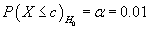Note that we reverse the sign to accommodate the fact that we are looking at a problem where the test statistic is lower than the expected value. If this trips you up, you can change the test statistic to, for instance, the number of seeds that do not germinate; you can then use the standard method of P(X > c). Using a table for the standard normal distribution, where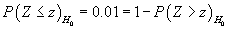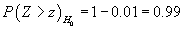we find that

z = –2.33

Note that we use the negative sign-depending on the type of table you use, the rationale for this choice may vary. Nevertheless, we are considering the left side (less than zero) of the standard normal distribution; as a result, the z value must be negative for any probability less than 0.5. Let's convert this z value to a critical value, c.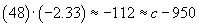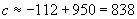Referring back to the problem, 821 (82%) of the seeds from a given packet germinate. Because 821 < 838, we reject the null hypothesis, and we proceed on the assumption that the germination rate of the seeds is less than 95%.

Let's quickly consider the alternative approach mentioned earlier. We'll look at the number of seeds that do not germinate. Our hypotheses remain the same, but our test statistic changes to the following:

X = The number of seeds out of 1,000 that do not germinate

We know that the mean number of seeds that germinate out of 1,000 is 950; thus, the mean number of seeds that do not germinate is 50. The standard deviation of 48 applies in both cases: 902 to 998 seeds germinate, 2 to 98 seeds to not (these numbers are obtained by adding and subtracting the standard deviation from the mean in each case). Again, we'll use α = 0.01. Then,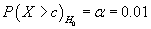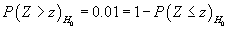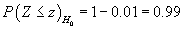Using the table of values,

z = 2.33

Let's calculate c using this z value, where the mean of X is 50 and the standard deviation is 48.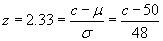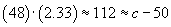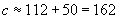Because the question states that only 179 seeds do not germinate, and because 179 > 162, we are justified in rejecting the null hypothesis.

Practice Problem: What is the probability that the null hypothesis was incorrectly rejected in the above problem?

Solution: The probability that the null hypothesis was incorrectly rejected is the value of α that we chose: 0.01, or 1%. The problem can be legitimately approached using a different α value--whatever value you chose, this is the probability of getting a false negative (that is, the probability that the hypothesis test led to an incorrect rejection of the null hypothesis).